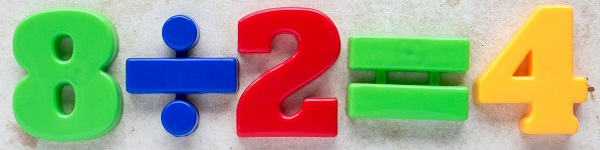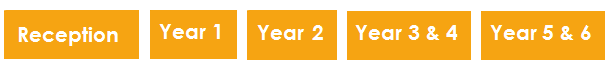School year > Subject guides > Maths in Year 2

# Maths in Year 2 (age 6–7)At this age, here are some things your child is likely to be doing:

• Comparing and ordering numbers from 0 to 100, using <, > and = signs
• Counting in steps of 2, 3 and 5 from 0, and in tens from any number, forward and backward
• Using place value and number facts to solve problems
• Adding and subtracting two-digit numbers using mental and written methods
• Recalling and using addition and subtraction facts up to 20, and deriving related facts up to 100
• Recalling and using multiplication and division facts for the 2, 5 and 10 multiplication tables, and recognising odd and even numbers
• Solving addition and subtraction money problems, using symbols for pounds and pence
• Telling and writing the time to the nearest five minutes
• Identifying, describing, comparing and sorting 2D and 3D shapes
• Interpreting and constructing pictograms, tally charts, block diagrams and simple tables

### Different ways to teach and learn maths at school

Maths will normally be taught on a daily basis, in a timetabled lesson, but children practise and use their maths throughout the school day.

From the outset, there will be a lot of focus on developing basic maths skills, such as remembering basic addition and subtraction facts, for example, 9 – 6 and 4 + 6. Teachers often set aside part of each lesson to devote to this learning.

### Assessment

Assessment for young children is informal and discrete and helps to inform the teacher’s planning so they can support each child in their class. In Year 2, children will be assessed on reading, mathematics and English grammar, punctuation and spelling. These tests will be marked by the teachers in your child's school.

Find out more about the Key Stage 1 SATs >